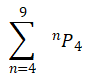## [LATEST]\$type=sticky\$show=home\$rm=0\$va=0\$count=4\$va=0

Permutations and Combinations is one of the most important chapters of Algebra in the JEE syllabus and other engineering exams. For JEE Mains, it has 4% weightage and for JEE Advanced, it has 5% weightage..

Q1. The number of ways in which we can get a score of 11 by throwing three dice is
•  18
•  27
•  45
•  56
Solution
Dice is marked with numbers 1, 2, 3, 4, 5, 6. If the sum of dice in three throws is 11, then observations must be 1, 4, 6;…1, 5, 5;…2, 3, 6;…2, 4, 5;… 3, 3, 5; …3, 4, 4
We can get this observation in 3! + 3!/2! + 3! + 3! + 3!/2! + 3!/2! = 27 ways

Q2.There are two bags each containing balls. If a man has to select equals number of balls from both the bags the number of ways in which he can do so if he must chose at least one ball from each bag is
•  m2
•  2mCm
•  2mCm - 1
•  None of these
Solution
The number of ways of selecting r(0 ≤ r ≤ m) balls out of m is mCr.
Therefore, the number of ways if selecting balls from each of the bag is (mCr)2, Further the number of ways of selecting equal number of balls from each of the two bags, choosing at least one from each bag, is
(mC1)2 + (mC2)2 + ... + (mCm)2 = 2mCm - 1
[∵ (mC0)2 + (mC1)2 + ... + (mCm)2 = 2mCm]

Q3.  Eight chairs are numbered 1 to 8. Two women and three men wish to occupy one chair each. First, the women choose the chairs from amongst the chairs marked 1 to 4, and then the men select the chairs from amongst the remaining. The number of possible arrangements is
•  6C3 x 4C2
•  4P2 x 4P3
•  4C2 + 4P3
•  None of these
Solution
Two women can choose two chairs out of 1, 2, 3, 4 in 4C2 ways, and can arrange among themselves in 2! ways. Three men can choose 3 chairs out of 6 remaining chairs in 6C3 ways and can arrange themselves in 3! ways.
Therefore, total number of possible arrangements is 4C2 X 2! X 6C3 X 3! = 4P2 X 6P3

Q4.  n is selected from the set {1,2,3,...,10} and the number 2n + 3n + 5n is formed. Total number of ways of selecting so that the formed number is divisible by 4 is equal to
•  50
•  49
•  48
•  None of these
Q5.Let A = {x1, x2, x3, ..., x7}, B = {y1, y2, y3}. The total number of functions f:A → B that are on to and there are exactly three element in such that f(x) = y2 is equal to
•  490
•  510
•  630
•  None of these
Solution
Three elements from set ‘A’ can be selected in 7C3 ways. Their image has to be Remaining 2 images can be y2assigned to remaining 4 pre-images in 24 ways. But the function is onto, hence the number of ways is 24 - 2. Then the total number of functions is 7C3 X 14 = 490

Q6. Number of ways in which 25 identical things be distributed among five persons if each gets odd number of things is
•  25C4
•  12C8
• 14C10
•  13C13
Q7.The number of integral solutions of x + y + z = 0 with x ≥-5, y≥-5,z≥-5, is
•  134
•  136
•  138
•  140
Solution
Let x = p, y = q - 5 and z = r - 5, where p,q,r ≥ 0
Then the given equation reduces to p + q + r = 15 -- (1)
Now, we have to find non-negative integral solution of Eq. (1). The total number of such solutions is 15+3-1C3-1 = 17C2 = 136

Q8.The total number of five-digit numbers of different digits in which the digit in the middle is the largest is
••  T33(3!)
•  30(3!)
•  None of these
Q9.The total number of ways in which persons can be divided into couples is
•  2n!/(n!n!)
•  2n!/(2!)n
•  2n!/(n!(2!)n)
•  None of the above
Solution
Here, we are dividing 2n people in group of 2 each, and we are concerned with mere grouping. Hence, the required number of ways is 2n!/(n!(2!)n)

Q10. In how many different ways can the first 12 natural numbers be divided into three different groups such that numbers in each group are in A.P.?
•  1
•  5
•  6
• 4
Solution
No group of four members from the first 12 natural number can have the common difference 4.
If one group including 1 is selected with the common difference 1, then the other two group can have the common difference 1 or 2.
If one group including 1 is selected with the common difference 2, then one of the other two groups can have the common difference 2 and the remaining group will have common difference 1.
If one group including 1 is selected with common difference 3, then the other two group can have the common difference 3 Therefore, the required number of ways is 2 + 1 + 1 = 4#### Written by: AUTHORNAME

AUTHORDESCRIPTION## Want to know more

Please fill in the details below:

## Latest NEET Articles\$type=three\$c=3\$author=hide\$comment=hide\$rm=hide\$date=hide\$snippet=hide

Name

ltr
item
BEST NEET COACHING CENTER | BEST IIT JEE COACHING INSTITUTE | BEST NEET & IIT JEE COACHING: Permutations and Combinations-Quiz-1
Permutations and Combinations-Quiz-1
https://1.bp.blogspot.com/-ZHSzfpa1RGE/YNyypljpenI/AAAAAAAAAwI/Cz-oOZEAa8QPIfkj_NdFmjQf2-jIsXlHQCLcBGAsYHQ/s400/PC.jpg
https://1.bp.blogspot.com/-ZHSzfpa1RGE/YNyypljpenI/AAAAAAAAAwI/Cz-oOZEAa8QPIfkj_NdFmjQf2-jIsXlHQCLcBGAsYHQ/s72-c/PC.jpg
BEST NEET COACHING CENTER | BEST IIT JEE COACHING INSTITUTE | BEST NEET & IIT JEE COACHING
https://www.cleariitmedical.com/2021/07/permutations-and-combinations-quiz-1.html
https://www.cleariitmedical.com/
https://www.cleariitmedical.com/
https://www.cleariitmedical.com/2021/07/permutations-and-combinations-quiz-1.html
true
7783647550433378923
UTF-8

STAY CONNECTED